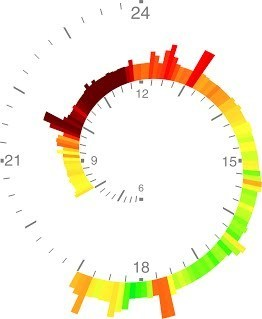# Spiral clock chart

Hi there guys i need to make a spiral clock chart like this onebut i have no idea how to start can you guys please give me some pointers

I would start with the wiki page on the Archimedean Spiral. Once you have read enough to know the mathematical formula you can get started. Try building a CGPath that describes as much of the spiral as you need, and then stroking the path.
To add radial strokes (hour markers etc.) you just need to start at the correct point (again given by the spiral formula) and move radially.
Get started and experiment, there will probably be more specific questions as you get started.

Got the spiral the way i wanted to be but i can add the hours
this was the code i used

• (UIBezierPath *)spiralPath {
int centerX = 160;
int centerY = 240;

int iDegrees = 15;
int iN = 360 / iDegrees;
double dAngleOne;
double dAngle;
double dSpace = 50;
double dSpaceStep;
double dR = 0;
double X = 0.0;
double Y = 0.0;
double iTurns = 1.2;

``````dAngleOne = M_PI * iDegrees / 180.0;
dSpaceStep = 0; // -dSpace / (double)iN;
double iCount = -1;

CGPoint c1 = CGPointMake(0, 0);
CGPoint c2 = CGPointMake(0, 0);
``````
``````UIBezierPath *path = [UIBezierPath bezierPath];

[path moveToPoint:CGPointMake(centerX, centerY)];

for (int k = 0; k < iTurns; k++)
{
for (int i = iDegrees; i <= 360; i += iDegrees)
{
dSpaceStep += dSpace / (double)iN;
dAngle = M_PI * i / 180.0;

// Get points.
iCount += 1;
if ((iCount == 0) || (iCount == 1))
{
// Control-point.
X = ((dR + dSpaceStep) / cos(dAngleOne)) * cos(dAngle) + centerX;
Y = ((dR + dSpaceStep) / cos(dAngleOne)) * sin(dAngle) + centerY;

if (iCount == 0)
c1 = CGPointMake(X, Y);
else
c2 = CGPointMake(X, Y);
}
else
{
// End-point.
X = (dR + dSpaceStep) * cos(dAngle) + centerX;
Y = (dR + dSpaceStep) * sin(dAngle) + centerY;
iCount = -1;
``````return path;